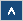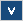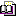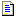Verilog Online HelpTable of ContentsBit-selectBlock StatementsBuilt-in PrimitivesCase StatementContinuous AssignmentsConversion FunctionsCommentsCompiler DirectivesConcatenationsConditional OperatorDelaysDisable StatementDisplay TasksEdge Sensitive PathExpression Bit LengthFile I/O FunctionsFunctionsIdentifiersIf StatementInteger ConstantsIntra-assignment Timing ControlsKeywordsLoop StatementsMemoriesmin:typ:max DelaysModule DeclarationModule InstantiationModule Path DeclarationModule Path PolarityNet Data TypesOperatorsParametersPart-selectPLA Modeling TasksProbabilistic Distribution FunctionsProcedural AssignmentsProcedural Continuous AssignmentsProcedural Timing ControlRange SpecificationReal ConstantsRegister Data TypesSimulation Control TasksSimulation Time FunctionsSpecify BlockState Dependent PathStochastic Analysis TasksStrengthsStringsStructured ProceduresTasksTimescale System TasksTiming Check TasksUDP DeclarationUDP InstantiationUDP State TableValue Change Dump (VCD) FileVectors

# Integer Constants

## Formal Definition

The integer constants are used to specify numbers.

## Simplified Syntax

sign size 'base number

## Description

Integer constants can be specified as unsized numbers (Example 1) or as sized numbers (Example 2). Sign, size and base are optional and they can be separated by white spaces. If none are used, then a number is positive and a decimal. Size should be a positive constant value (decimal number) and it specifies number of bits used for the integer. The base format should contain two characters: apostrophe (') and a base identifier. The base identifier and apostrophe cannot be separated by any white space. Legal base specifications are d and D for decimal numbers, h and H for hexadecimal numbers, o and O for octal numbers, b and B for binary numbers.

Decimal numbers can contain only digits and underscores (_). Binary numbers can contain only 0, 1, unknown values (x and X), high-impedance values (z and Z) and underscores. Each character in a binary number has 1 bit. Octal numbers can be specified using 0, 1, 2, 3, 4, 5, 6, 7, unknown values (x and X), high-impedance values (z and Z) and underscores. Each character in an octal number has a 3 bit equivalent. Legal characters for hexadecimal numbers are unknown values (x and X), high-impedance values (z and Z), digits (0, 1, 2, 3, 4, 5, 6, 7, 8, 9), letters (a, A, b, B, c, C, d, D, e, E, f, F) and underscores. Each character in a hexadecimal number has a 4 bit equivalent.

Decimal numbers without the base format and size specified are treated as signed integers, but decimal numbers with base format are treated as unsigned integers (see Arithmetic expressions with registers and integers for more details).

If a specified number is bigger than a specified size constant, then the most significant bits of numbers will be truncated. If number is smaller than the size constant, then it will be padded to the left with zeros. If the most significant bit of a specified number has an unknown (x) or high-impedance (z) value, then that value will be used to pad to the left (Example 3).

Underscore character (_) is ignored and can be used to enhance readability. It cannot be the first character in number.

The question mark (?) can be used in specifying numbers with high-impedance value (z) and is very useful in case statements.

## Examples

Example 1

15
'h f
'o 17
'd 15
'b 1111
'b 1_1_1_1

All the above numbers are equal. The first number is a decimal number without the base specified, the second one is a hexadecimal number, the third is an octal number, the fourth is a decimal number with base specified, and the fifth and sixth are binary numbers.

Example 2

-5'b1_1011
10 'd 20
8'h z
6'o 71

Examples of sized integers.

Example 3

8'b0 is equal to 8'b00000000
8'b1 is equal to 8'b00000001
8'bz is equal to 8'bzzzzzzzz
8'bx is equal to 8'bxxxxxxxx

Examples of padding to the left.

## Important Notes

• Decimal numbers without specified size and base format are treated as signed integers.

• Decimal numbers with the base format specified are treated as unsigned integers.